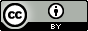Proving the limit laws

Addition. Let $$E\subset \mathbf{R}$$ be a subset of the real numbers, $$f, g : E \to \mathbf{R}$$ be real functions, and $$L_1, L_2\in \mathbf{R}$$ be real numbers. Now suppose $$\lim_{x\to a} f(x) = L_1$$ and $$\lim_{x\to a} g(x) = L_2$$. We would like to show that $$\lim_{x\to a} (f(x)+g(x)) = L_1+L_2$$.

Since $$\lim_{x\to a} f(x) = L_1$$ and $$\lim_{x\to a} g(x) = L_2$$, we know that $\forall \epsilon >0 \exists \delta >0 \forall x (0 < |x-a|<\delta \to |f(x)-L_1|<\epsilon)$ and $\forall \epsilon >0 \exists \delta >0 \forall x (0 < |x-a|<\delta \to |g(x)-L_2|<\epsilon)$ Now let $$\epsilon > 0$$. We need to find $$\delta > 0$$ such that $0<|x-a|<\delta \to |(f(x)+g(x))-(L_1+L_2)|<\epsilon$ But \begin{align} |f(x)+g(x)-(L_1+L_2)| &= |f(x)-L_1 + g(x)-L_2| \\ &\leq |f(x)-L_1| + |g(x)-L_2| \end{align} by the triangle inequality. So if we can show $$|f(x)-L_1| + |g(x)-L_2|<\epsilon$$, then our result follows immediately. But, $$\frac{\epsilon}{2}$$ being a positive real number, we have $$\delta_1,\delta_2 > 0$$ such that $0 < |x-a|<\delta_1 \to |f(x)-L_1|<\frac{\epsilon}{2} \\ 0 < |x-a|<\delta_2 \to |f(x)-L_2|<\frac{\epsilon}{2}$ So if we let $$\delta := \min(\delta_1,\delta_2)$$, then $$|f(x)-L_1|$$ and $$|g(x)-L_2|$$ are both less than $$\frac{\epsilon}{2}$$, so $|f(x)-L_1| + |g(x)-L_2|< \frac{\epsilon}{2} + \frac{\epsilon}{2} = \epsilon$ which completes the proof. $$\square$$